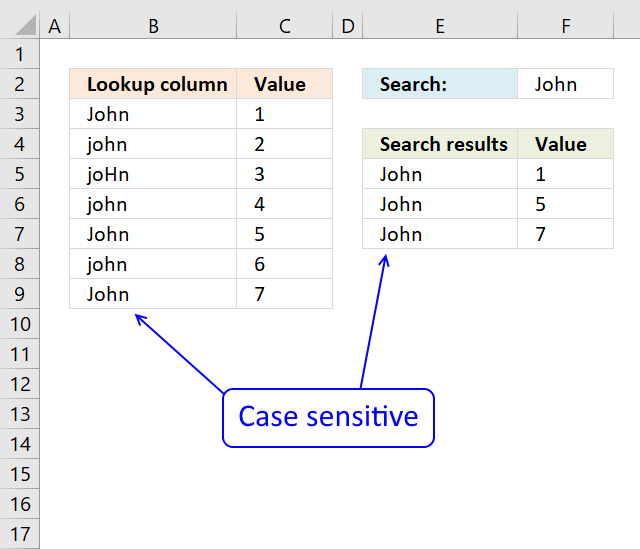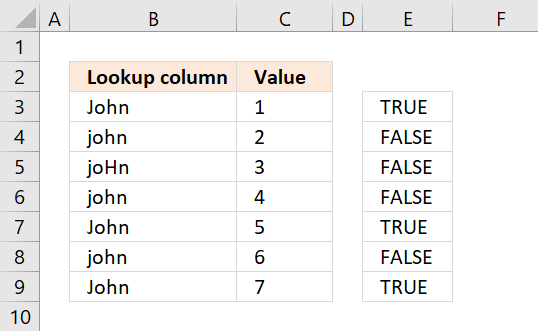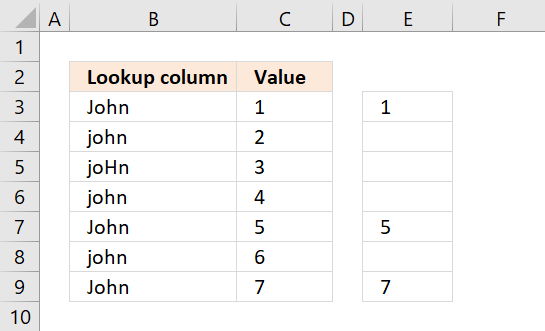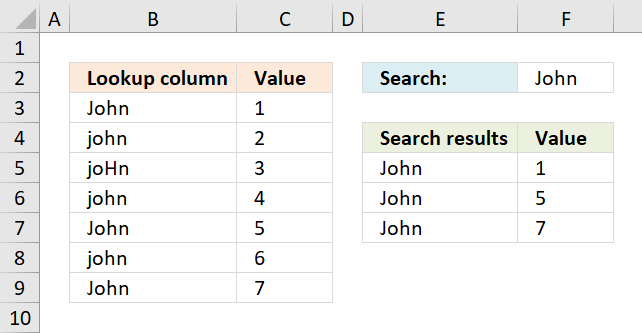Author: Oscar Cronquist Article last updated on February 12, 2018The array formula in cell F5 returns adjacent values from column C where values in column B matches the search value in cell F2 (case sensitive).

Array formula in E5:

=INDEX(\$B\$3:\$B\$9, SMALL(IF(EXACT(\$B\$3:\$B\$9, \$F\$2), MATCH(ROW(\$B\$3:\$B\$9), ROW(\$B\$3:\$B\$9)), ""), ROWS(\$A\$1:A1)))

Array formula in F5:

=INDEX(\$C\$3:\$C\$9, SMALL(IF(EXACT(\$B\$3:\$B\$9, \$F\$2), MATCH(ROW(\$B\$3:\$B\$9), ROW(\$B\$3:\$B\$9)), ""), ROWS(\$A\$1:A1)))

### How to enter an array formula

Double press with left mouse button on cell E5, copy above formula and paste to cell E5. Press and hold CTRL + SHIFT, then press Enter once. Release all keys.

The formula bar displays the formula enclosed with curly brackets, they appear automatically. Don't enter these values yourself.

Repeat above steps to enter array formula in cell F5. Then copy cell range E5:F5 and paste to cells below as far as needed.

### Explaining formula in cell E5

#### Step 1 - Check if values are an exact match to lookup value

EXACT(\$B\$3:\$B\$9, \$F\$2)

becomes

EXACT({"John";"john";"joHn";"john";"John";"john";"John"},"John")

and returns {TRUE; FALSE; FALSE; FALSE; TRUE; FALSE; TRUE}

The picture below shows the array in column E. TRUE means that the value in column B on the same row is identical to the lookup value.#### Step 2 - Convert boolean array to row numbers if TRUE

IF({TRUE; FALSE; FALSE; FALSE; TRUE; FALSE; TRUE},MATCH(ROW(\$B\$3:\$B\$9), ROW(\$B\$3:\$B\$9)), "")

becomes

IF({TRUE; FALSE; FALSE; FALSE; TRUE; FALSE; TRUE},{1;2;3;4;5;6;7}, "")

and returns {1;"";"";"";5;"";7}.

The picture below displays the array in column E. Now we know which rows the identical values have.#### Step 3 - Extract the k-th smallest value from array

SMALL(IF(EXACT(\$B\$3:\$B\$9, \$F\$2),MATCH(ROW(\$B\$3:\$B\$9), ROW(\$B\$3:\$B\$9)), ""), ROWS(\$A\$1:A1))

becomes

SMALL( {1;"";"";"";5;"";7}, ROWS(\$A\$1:A1))

becomes

SMALL( {1;"";"";"";5;"";7}, 1)

and returns 1. Note that ROWS(\$A\$1:A1) change when you copy the cell and paste to cells below. This makes the formula show all identical values.

#### Step 4 - Get value from cell range

INDEX(\$B\$3:\$B\$9, SMALL(IF(EXACT(\$B\$3:\$B\$9, \$I\$2), MATCH(ROW(\$B\$3:\$B\$9), ROW(\$B\$3:\$B\$9)), ""), ROWS(\$A\$1:A1)))

becomes

INDEX(\$B\$3:\$B\$9, 1)

becomes

INDEX({"John";"john";"joHn";"john";"John";"john";"John"}, 1)

and returns John in cell E5.### Get excel *.xlsx file

Case sensitive vlookup and returning multiple values

EXACT(text1, text2)
Checks whether two text strings are exactly the same and returns TRUE or FALSE. EXACT is case sensitive.

IF(logical_test,[value_if_true], [value_if_false])
Checks whether a condition is met, and returns one value if TRUE, and another value if FALSE

SMALL(array,k) returns the k-th smallest row number in this data set.

INDEX(array,row_num,[column_num])
Returns a value or reference of the cell at the intersection of a particular row and column, in a given range

MIN(number1,[number2])
Returns the smallest number in a set of values. Ignores logical values and text

ROW(reference) returns the rownumber of a reference